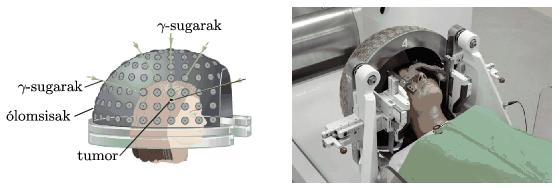Mathematical and Physical Journal
for High Schools
Issued by the MATFUND Foundation
 Already signed up? New to KöMaL?

#Problem P. 5120. (March 2019)

P. 5120. When radiotherapy is applied, the tumour is exposed to a certain dose (absorbed energy per unit mass) of radiation, such that the surrounding healthy tissues do not absorb a too large dose. Investigate this problem by using the following simple model: the patient's head is considered to be a uniform sphere of radius 8 cm. The small tumour is located at the centre of the sphere and is radiated by beams of $\displaystyle \gamma$ photons, having the same intensity. The radiation beams are aimed from the direction of five different diameters. The intensity (power per unit area) of the radiation beam is decreasing exponentially as the beam passes the tissues in the sphere, in accordance with the following equation: $\displaystyle I(x)=I_0{\rm e}^{-\mu x}$.(The distance of $\displaystyle x_0=\ln 2/\mu$ is called the half-value layer; its numerical value depends on the energy of the photons and the material of the absorbing medium. E.g. for photons of energy 2.5 MeV it is $\displaystyle x_0=23$ cm in water.)Two different types of radiation can be applied: either $\displaystyle \gamma$-photons of energy 1 MeV emitted by a source of $\displaystyle ^{60}\rm Co$, for which $\displaystyle \mu=0.07~\rm cm^{-1}$, or $\displaystyle \gamma$-photons of energy 6 MeV, which can be generated by a particle accelerator, here $\displaystyle \mu=0.028~\rm cm^{-1}$.

Which radiation spares more the healthy tissues, that is, which one results a smaller dose at the surface of the sphere? What is the value of the dose next to the surface of the sphere if the necessary dose value at the tumour is $\displaystyle D$?

(5 pont)

Deadline expired on April 10, 2019.

Sorry, the solution is available only in Hungarian. Google translation

Megoldás. Az elnyelt dózis a sugárzás intenzitásával arányos. A daganat (tumor) helyén érvényes $\displaystyle D$ dózis és a felület közelében figyelembe vehető $\displaystyle D_0$ dózis közötti kapcsolat (öt nyaláb alkalmazásával):

$\displaystyle D=5D_0\,{\rm e}^{-\mu\cdot (8~{\rm cm})},$

vagyis

$\displaystyle D_0=\frac{D}{5}{\rm e}^{\mu\cdot (8~{\rm cm})}.$

A megadott $\displaystyle \mu$ értékekkel számolva az 1 MeV-es $\displaystyle \gamma$-fotonok esetében a $\displaystyle D_0=0{,}35\,D$, 6 MeV-es $\displaystyle \gamma$-fotonokkal pedig $\displaystyle D_0=0{,}25\,D$ eredményt kapjuk. Látható, hogy a nagyobb energiájú gamma-sugárzás – a nagyobb felező rétegvastagsága miatt – kisebb károsodást okoz az egészséges szöveteknél, mint a kisebb energiájú (de jobban gyengülő) gamma-sugárzás.

### Statistics:

 20 students sent a solution. 5 points: Andorfi István, Czett Mátyás, Debreczeni Tibor, Markó Gábor, Pácsonyi Péter, Tiefenbeck Flórián, Varga Vázsony. 4 points: Fülöp Sámuel Sihombing, Kozák 023 Áron, Mácsai Dániel, Makovsky Mihály, Németh Csaba Tibor, Olosz Adél. 3 points: 4 students. 2 points: 3 students.

Problems in Physics of KöMaL, March 2019